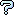All about flooble | fun stuff | Get a free chatterbox | Free JavaScript | Avatarsperplexus dot infoEqual Tangents (Posted on 2010-10-03)C(UV) denotes the circle with diameter UV.

T(P,QR) denotes the tangential distance |PS|,
where point P lies outside C(QR), point S
lies on C(QR), and PS is tangent to C(QR).

Let A, B, C, and D be distinct, collinear
points in that order.

Construct a point E on line AD such that
|EF| = T(E,AB) = T(E,CD) = |EG|

 Submitted by Bractals Rating: 3.0000 (1 votes) Solution: (Hide) Construction: Construct circles C(AB) and C(CD). Draw a circle K1 intersecting circle C(AB) in points P1 and Q1 and circle C(CD) in points R1 and S1. Let T1 be the intersection of lines P1Q1 and R1S1. Construct the point T2 similarly. The intersection of lines T1T2 and AD is the desired point E. Note: When I say construct a circle, I mean that only one circle is possible from the information given; whereas, when I say draw a circle, I mean that an infinite number of circles are possible from the infomation given. Proof: T(X,YZ) is the square root of the "power" of point X with respect to circle C(YZ). The locus of points E where T(E,AB) = T(E,CD) is the "radical line" of the circles C(AB) and C(CD). The radical line of circles K1 and C(AB) is the line P1Q1. The radical line of circles K1 and C(CD) is the line R1S1. The point T1 lies on the radical line of circles C(AB) and C(CD) (see "radical center"). Similarly, T2 lies on the radical line of circles C(AB) and C(CD). Therefore, T1T2 is the radical line of circles C(AB) and C(CD). Thus, E is the desired point. Note: See Larry's post for an analytic calculation of the distance from A to E. This distance is constructible and therefore is an alternate method for constructing point E.Comments: ( You must be logged in to post comments.)
 Subject Author Datere(3): Construction Charlie 2010-10-05 01:05:46re: Algebraic solution leads to geometric Larry 2010-10-04 22:34:19re(2): Construction brianjn 2010-10-04 21:13:39re(2): Does this work? broll 2010-10-04 12:20:04re: Does this work? Charlie 2010-10-04 12:16:49re: Construction Charlie 2010-10-04 12:05:22Does this work? broll 2010-10-04 02:53:11Construction brianjn 2010-10-04 02:20:35No Subject Ady TZIDON 2010-10-03 15:40:05re: Algebraic solution Bractals 2010-10-03 14:39:12Algebraic solution Larry 2010-10-03 14:14:53Please log in:

 Search: Search body:
Forums (0)